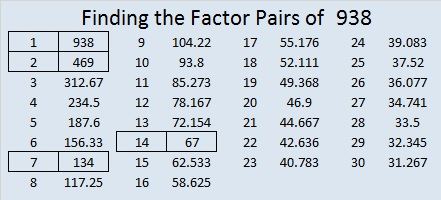# 938 and Level 5

Can you figure out where the factors 1 – 10 go in the first column and top row so that this level 5 puzzle will function as a multiplication table?Print the puzzles or type the solution on this excel file: 10-factors-932-941

Now I’ll share a few facts about the number 938.

938 is a palindrome in two consecutive bases:
It’s 343 in BASE 17 because 3(17²) + 4(17¹) + 3(17º) = 938
It’s 2G2 in BASE 18 (G is 16 base 10), because 2(18²) + 16(18¹) + 2(18º) = 938

• 938 is a composite number.
• Prime factorization: 938 = 2 × 7 × 67
• The exponents in the prime factorization are 1, 1, and 1. Adding one to each and multiplying we get (1 + 1)(1 + 1)(1 + 1) = 2 × 2 × 2 = 8. Therefore 938 has exactly 8 factors.
• Factors of 938: 1, 2, 7, 14, 67, 134, 469, 938
• Factor pairs: 938 = 1 × 938, 2 × 469, 7 × 134, or 14 × 67
• 938 has no square factors that allow its square root to be simplified. √938 ≈ 30.62678566This site uses Akismet to reduce spam. Learn how your comment data is processed.